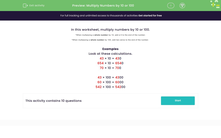# Multiply Numbers by 10 or 100

In this worksheet, students multiply numbers by 10 or 100.Key stage:  KS 2

Curriculum topic:   Number: Number and Place Value

Curriculum subtopic:   Count in Multiples (4, 8, 50 and 100)

Difficulty level:#### Worksheet Overview

In this activity, we will be multiplying numbers by 10 or 100.

To multiply a whole number by 10: move each digit one place to the left, then fill any empty places with zero.

43 × 10 = 430

654 × 10 = 6540

70 × 10 = 700

To multiply a whole number by 100: move each digit two places to the left, then fill any empty places with zero.

43 × 100 = 4300

60 × 100 = 6000

542 × 100 = 54200

Let's try some examples together.

Example 1

Multiply 65 by 10.

To multiply by 10, each digit moves one place to the left.

In 65, we have 6 tens and 5 ones.

The 6 tens becomes worth 6 hundreds.

The 5 ones becomes worth 5 tens

We fill the ones place with zero as a placeholder.

65 × 10 = 650

Example 2

Multiply 24 by 100.

To multiply by 100, each digit moves two places to the left.

In 24, we have 2 tens and 4 ones.

The 2 tens becomes worth 2 thousands.

The 4 ones becomes worth 4 hundreds.

We fill the ones and tens places with zeros as placeholders.

24 × 100 = 2400

Now that you know how to multiply by 10 and 100, you are ready to try the next 10 questions.

### What is EdPlace?

We're your National Curriculum aligned online education content provider helping each child succeed in English, maths and science from year 1 to GCSE. With an EdPlace account you’ll be able to track and measure progress, helping each child achieve their best. We build confidence and attainment by personalising each child’s learning at a level that suits them.

Get started